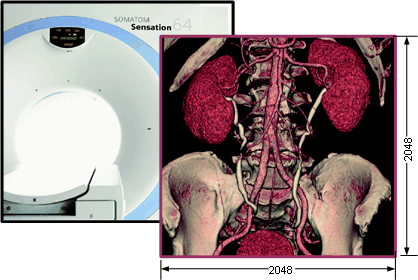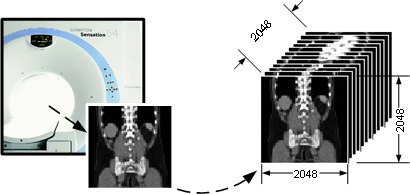# 5 Implementation

## 5.1Introduction

Modern medical imaging devices provide ultra high-resolution data from the patient that is crucial for diagnosis. An example is Siemens Somatom (Figure 5‑1), which can produce informative 20483 high resolution data. As the resolution increases, the data processing requirements increase as well. Real time interactive frame rates can be achieved at 33 Hz. minimum. This means 33 ´ 20483 data cells should be visited every second and processed within a number of algorithms (Figure 5‑2). Such a speed is impossible to implement with software and conventional hardware. Special purpose hardware should be used as described in chapter 1.Figure 5‑1: Medical data obtained from CT scanner.Figure 5‑2: Reconstruction of three-dimensional data with high resolution.

A visualization process consists of three stages, that are, ray tracing, surface normal estimation, and shading. The latter two are applied to the final image obtained from the former. Therefore, the main part of this time-consuming process is ray tracing which is to be implemented with DSPs. Source code for this stage is given in next section.

## 5.2Source Code

The C code for ray tracing is given below. VOX stands for voxel, the 3D data element which is represented by a coordinate triple such as (x, y, z) and its structure is as follows:

`struct VOX`
`{`
`short x;`
`short y;`
`short z;`
`}`

TEMP is the structure which holds ray template as explained in previous chapters. Every element of the template defines the difference on related axis that is necessary to move from one coordinate to the other in 3D discrete data space.

`struct TEMP`
`{`
`int u_diff;`
`int v_diff;`
`int n_diff;`
`}`

BASEWINDOW is the structure to hold the edge coordinates of the view plane (Figure 5‑3).

`struct BASEWINDOW`
`{`
`VOX leftdown;`
`VOX leftup;`
`VOX rightdown;`
`VOX rightup;`
`}`Figure 5‑3: Structure BASEWINDOW and its relation with the view plane.

The variable “wcs_data” at line 39 holds the resolution of the dataset. The variable “vpw_data” at line 24 holds the 2D resolution of the view plane, that are the width and height of the plane.

Given the data with 3D resolution as (xres, yres, zres), variable U at line 31 and variable V at line 29 holds xres,  and yres respectively. Variable k in line 34 stands for zres. It can be seen from the source code that every data element within the dataset is visited. Thus the loop between lines 29 and 55 runs xres´yres´zres times which can be stated as the heart of the code.

The code between line 11 and 23 creates a ray template and loads to the memory, regarding to the projection specifications. This is achieved by the function “CreateRayTemplate”.

View plane is defined in a coordinate system, which is different from the data space. View plane is functioning as a camera. The data space is called “World Coordinate System (WC)” and the view plane space is called “View Reference Coordinate System (VRC)”. Since the edge coordinates of the view plane are defined in VRC within the structure BASEWINDOW, it needs to be transformed into WC prior to tracing process. This is achieved by the function “TransformVRCtoWC” at line 33.

` `
`int  RayTrace(BYTE * pdata)`
`{`
`1.                short axis, sayac, voxSayac, dU, dV;`
`2.                int U,V,numb_temp,k,val; `
`3.                int height=1; int width=1; int add;                                                   `
`4.                long offset, voxToplam;`
`5.                VOX vox, voxTmp, * fpCOOR;`
`6.                BYTE RGB, voxColor, * fpRGB; `
`7.                TEMP *temp;`
`8.                BASEWINDOW  Base;`
`9.                FILE * fpCOORraw, * fpRGBraw; `
`10.            int i,j;`
` `
`11.            axis=FindAxis();`
`12.            basewdw=CalculateBaseWindow(axis);                                                    `
`13.            CreateRayTemplate();`
`14.            rewind(fp_ray_temp);`
`15.            fseek(fp_ray_temp,0,SEEK_END);`
`16.            offset=ftell(fp_ray_temp);`
`17.            numb_temp=offset/(3*sizeof(int));`
`18.            temp=(TEMP *) malloc(12 *  prwdw.time.ray_template);`
`19.            rewind(fp_ray_temp);`
`20.            for(k=0 ; k<prwdw.time.ray_template ; ++k)`
`21.            {`
`      fread(&temp[k],12,1,fp_ray_temp);`
`22.            }`
`23.            fclose(fp_ray_temp);`
`24.            dU=vpw_data.u_max - vpw_data.u_min;`
`25.            dV=vpw_data.v_max - vpw_data.v_min;`
`26.            fpbuf=(VOX *) calloc( dU * dV , sizeof(VOX) );`
`27.            fpCOOR=(VOX *) calloc( dU * dV , sizeof(VOX) );`
`28.            fpRGB=(BYTE *) calloc( dU * dV , sizeof(BYTE) );`
`29.            for(V=0 ; V < dV; ++V)`
`30.            { `
`31.                        for(U=0 ; U < dU ; ++U)`
`32.                        { `
`33.                                    voxTmp=TransformVRCtoWC(Base.x+U, Base.y+V, Base.z);`
`34.                                    for(k=0 ; k < numb_temp ; ++k)`
`35.                                    {`
`36.                                                ray_diff=temp[k];`
`37.                                                vox = voxTmp + ray_diff;`
`38.                                                voxTmp = vox;`
`39.                                                if(vox.x >= 0 && vox.y >= 0 && vox.z >= 0 `
`                   && vox.x  < wcs_data.x && vox.y  < wcs_data.y &&                  `
`                   vox.z < wcs_data.z)`
`40.                                                {`
`41.                                                            val=calculate_address(vox.x,vox.y,vox.z);`
`42.                                                            if (val <0 or val > (totalfilesizeinbytes-3))break;`
`43.                                                            if( file[val] > treshold)`
`44.                                                            {`
`45.                                                                        RGB=file[val];`
`46.                                                                        pdata[CalculateOffsetIn256Bmp(U,V,dU)]=RGB;`
`47.                                                                        k=numb_temp+1; `
`48.                                                                        fpCOOR[U+(dU*V)]= vox; `
`49.                                                                        fpRGB[U+(dU*V)]= RGB;`
`50.                                                                        fpbuf[U+(dU*V)]=TransformWCtoVRC(vox.x,vox.y,vox.z);`
`51.                                                            } `
`52.                                                }  `
`53.                                    } `
`54.                        } `
`55.            } `
`56.            free(temp);`
`57.            fpCOORraw=fopen("coorRT.tmp","w+b");`
`58.            fpRGBraw=fopen("rgbRT.tmp","w+b");`
`59.            for (i=0;i<(dU*dV);++i)`
`60.            {`
`61.                        fwrite(&fpCOOR[i],6L,1,fpCOORraw);`
`62.                        fwrite(&fpRGB[i],1L,1,fpRGBraw);`
`63.            }`
`64.            fclose(fpCOORraw);free(fpCOOR);`
`65.            fclose(fpRGBraw);free(fpRGB);`
`66.            return SUCCESS;`
`67.            }`

# References

           Levoy, M. (1988). Display of  Surfaces from Volume Data. Computer Graphics and Applications, 8(5), 29-37.

           Knittel, G. (1993). VERVE-Voxel Engine for Real-Time Visualization and Examination. Eurographics ‘93, 12(3), 37- 48

           Günther, T., Poliwoda, C., Reinhart, C., Hesser, J., and Manner, J. (1994). VIRIM: A Massively Parallel Processor for Real-Time Volume Visualization in Medicine. Proceedings of the 9th Eurographics Hardware Workshop,    103-108

           Boer, M.De, Gröpl, A., Hesser, J., and Manner, R. (1996). Latency-and Hazard-free Volume Memory Architecture for Direct Volume Rendering. Eurographics Workshop on Graphics Hardware, 109-119

           Osborne, R., Pfister, H., Lauer, H., McKenzie, N., Gibson, S., Hiatt, W., and Ohkami, T. (1997). EM-Cube: An Architecture for Low-Cost Real-Time Volume Rendering. Proc. Eurographics/SIGGRAPH Workshop on Graphics Hardware, 131-138

           Dachille, F. (1997). Volume Visualization Algorithms and Architectures. Research Proficiency Exam, SUNY at Stony Brook

           Bakalash, R.,  Pfister, H., Kaufmann, A., and Pacheco, R. (1992). Cube-2: An Extended Volume Visualization System for Arbitrary Parallel Projection. In Proceedings of the 1992 Eurographics Workshop on Graphics Hardware Cambridge UK.

           Pfister, H., Kaufman, A., and Chiueh, T. (1994). Cube-3: A Real-Time Architecture for High-Resolution Volume Visualization. 1994 Workshop on Volume Visualization , 75-83

           Pfister, H., and Kaufman, A. (1995).  Cube-4 : A scalable Architecture for Real-Time Volume Rendering. Technical Report TR-950115, Computer Science Department, SUNY at Stony Brook

         Kanus, U., Meissner, M., Strasser, W., Pfister, H., Kaufmann, A., Amerson, R., Carter, R.J., Culbertson, B., Kuekes, P., and Snider, G. (1997). Implementations of Cube-4 on the TERAMAC Custom Computing Machine. Computer and Graphics, 21(2), 199-208.

         Pfister, H., Hardenberg, J., Knittel, J., Lauer, H., and Seiler, L. (1999). The VolumePro Real-Time Ray-Casting System. SIGGRAPH 1999 Conference Proceedings, 251-260

         Texas Instruments TMS320C6713, TMS320C6713B Digital Signal Processors. Data sheet, Texas Instruments Inc.,  May 2004, Ver. SPRS1861